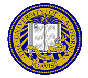# Homework 1 Revision 1

In problem 1, the formula for converting centigrade to Kelvin had a typo. The “−” should have been a “+”.

Due: October 6, 2021
Points: 100

In the given examples, what you type is in red and the program prints what is in black. Your program output should look exactly like the output in the examples, except that what you type won’t be in red.

1. (20 points) Two temperature scales are centigrade and Fahrenheit; a third, used much less commonly, is Kelvin. To convert a temperature from Fahrenheit to centigrade, use the following formula:
C = (5/9) * (F - 32)
where F is the temperature in Fahrenheit, and C is the temperature in centigrade. To convert from centigrade to Kelvin, use the following formula:
K = C + 273.15
where K is the temperature in Kelvin.

Write a program that prompts the user for a temperature in degrees Fahrenheit, and prints the corresponding centigrade and Kelvin temperatures. The temperature may be an integer or a real number. Round the temperatures to two places in the output.

Assume the input is valid; that is, the user will enter a real number.

To turn in: Please turn in the program in the file tempcvt.py.

Hint: The function to round a number x to two places is round(x, 2). So, to round x to two places, say round(x, 2).

Examples:

```
Enter temperature in degrees Fahrenheit: 3.14159
3.14 Fahrenheit is -16.03 centigrade and 257.12 Kelvin
```
```
Enter temperature in degrees Fahrenheit: -459.67
-459.67 Fahrenheit is -273.15 centigrade and -0.0 Kelvin
```
```
Enter temperature in degrees Fahrenheit: 212
212.0 Fahrenheit is 100.0 centigrade and 373.15 Kelvin
```

2. (35 points) Write a program to calculate the letter grade for an exam. The user will enter the points obtained on the exam and the total points possible. From this, determine and print the grade using the following rules:
1. A score of at least 90% is an A
2. A score at least 80% and up to but not including 90% is a B
3. A score at least 70% and up to but not including 80% is a C
4. A score at least 60% and up to but not including 70% is a D
5. A score less than 60% is an F

Prompt the user for the score and the total points possible. Reject any score that is greater than the total.

Assume the score and total are entered as integers.

To turn in: Please turn in the program in the file grade.py.

Hint: Use the function to round x to the nearest integer like this: round(x).

Examples:

```
Enter score: 160
Total possible: 200
A score of 160 out of 200 is a B
```
```
Enter score: 72
Total possible: 200
A score of 72 out of 200 is an F
```
```
Enter score: 90
Total possible: 121
A score of 90 out of 121 is a C
```
```
Enter score: 75
Total possible: 50
The score 75 is greater than the total possible, 50
```
3. (45 points) A paper1 proposed the following equation for the cumulative deaths for COVID-19 after x days, where x ≥ 30:
9 × 10-9 x6 − 6 × 10-6 x5 + 0.0015x4 − 0.1376 x3 + 7.4558 x2 − 97.284 x
Write a program that reads an integer number of days and prints the cumulative deaths. If the number of days is less than 18, reject the input. Round the number of deaths to the nearest integer.

To turn in: Please turn in the program in the file cd.py.

Examples:

```
Number of days: 150
After 150 days, the number of deaths predicted is 95029
```
```
Number of days: 30
After 30 days, the number of deaths predicted is 1152
```
```
Number of days: 8
The number of days, which was input as 8 must be at least 18
```

### Extra Credit

1. (10 points) Add exception handling to the answer for problem 1, so that if the input is not a number, the program prints an error message. In the second example, notice there are two decimal points (which is not allowed).

To turn in: Please turn in the program in the file tempcvt-ex.py.

Examples:

```
Enter temperature in degrees Fahrenheit: hello
The input must be a real number
```
```
Enter temperature in degrees Fahrenheit: 100..5
The input must be a real number
```

### Footnote

1. H. Kumar, P. Arora, M. Pant, A. Kumar, and S. Khan, “A simple mathematical model to predict and validate the spread of Covid-19 in India,” Materials Today: Proceedings, doi: 10.1016/j.matpr.2021.03.434Matt Bishop Office: 2209 Watershed Sciences Phone: +1 (530) 752-8060 Email: mabishop@ucdavis.edu
ECS 235A, Computer and Information Security
Version of September 27, 2021 at 2:05PM